## 3.2.4 Module COLIBR

This module is similar to the general module COLIB2, but the data is entered by "cards".

• dimension array M (in the blank common)
• call INITI
• call the module

CALL COLIBR (M,NFNOPO,NINOPO)

where

• M is the super array
• NF(NI)NOPO is the support number of the O.D.S. NOPO and its level

and as data "cards":

• a card containing 6 integer values, where:
• NBS is the number of vertices in the coarse mesh,
• NA is the number of edges in the coarse mesh,
• NBGRO is the number of blocks (or elements) in the coarse mesh,
• IMPRE is the print parameter
• NBFR is the number of faces to describe in order to assign a non-zero reference number to them,
• NDIMS is the dimension of the output space, if NDIMS = 2, side Z is ignored (without control); this allows us to create segments, triangles or quadrilaterals in the R˛ space,
• NBS cards containing the description of the coarse vertices, where:
• I is the number of the coarse vertex
• NOREF is its reference
• XYZ(3,I) its 3 coordinates
• NA cards containing the description of the coarse edges, where:
• I is the number of the coarse edge
• NS1 is the number of the vertex which is the origin of the edge
• NS2 is the number of its end-point
• IDEC is the splitting code of the edge: 0 for a straight edge split automatically into segments of the same length; 1 if we input the intermediate points (see XYZINT)
• NPC is the number of control points to be created on the edge (end-points excluded)
• NOREF is the edge reference number
XYZINT(3,.) are the coordinates of the control points of the edges given. If IDEC = 1, we enter these values as follows: XYZINT(J,K) is coordinate J of point K of the edge, where K = 1, NPC

• NBFR cards containing the description of the coarse faces, where:
• NOREF is the reference number of the face described
• NCGE is its geometric code (3: triangle, 4: quadrilateral)
• LISTE(1:NCGE) is the list of its vertices
• NBGRO cards containing the description of the coarse blocks, where:
• I is the number of the coarse element or block
• NCGE is the geometric type of the block (2: segment, 3: triangle,
4: quadrilateral, 5: tetrahedron, 6: pentahedron, 7: hexahedron)
• LIST(1,.) is the list of its vertices
• NCGED is the geometric type of the finite elements resulting from the splitting of the block (see remark below)
• NDSDE is the sub-domain number of the block (therefore that of all the sub-elements created)

Remark:

• In the present version NCGED = NCGE.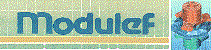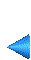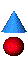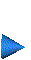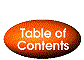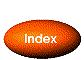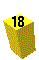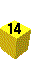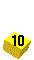Next: 3.2.5 Preprocessor COLIXX Up: 3.2 Multi-block method Prev: 3.2.3 Calling of the derived modules Index Contents